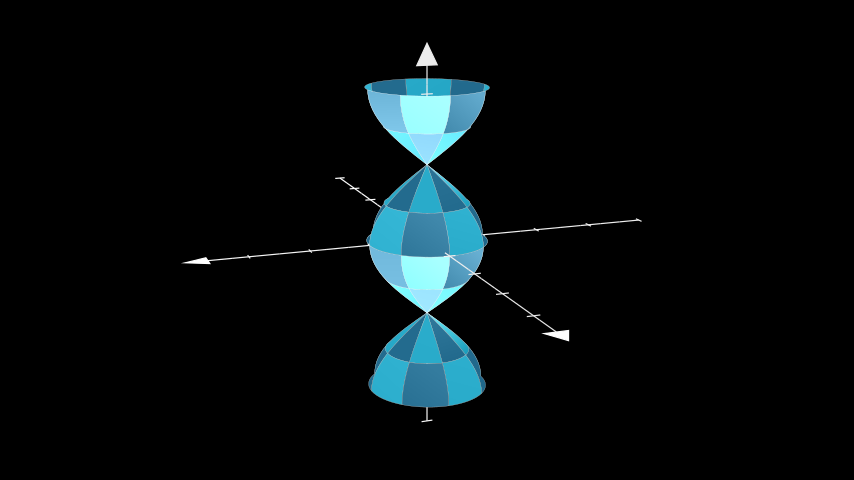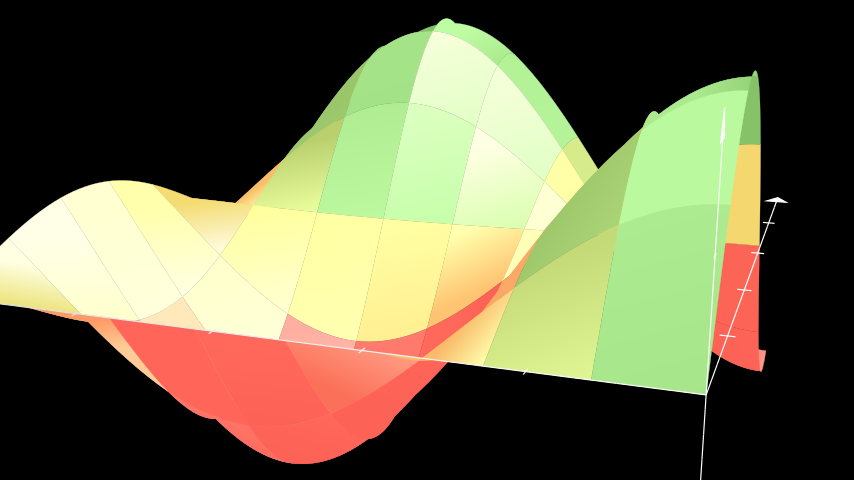# Surface#

Qualified name: `manim.mobject.three\_d.three\_dimensions.Surface`

class Surface(func, u_range=[0, 1], v_range=[0, 1], resolution=32, surface_piece_config={}, fill_color='#29ABCA', fill_opacity=1.0, checkerboard_colors=['#29ABCA', '#236B8E'], stroke_color='#BBBBBB', stroke_width=0.5, should_make_jagged=False, pre_function_handle_to_anchor_scale_factor=1e-05, **kwargs)[source]#

Bases: `VGroup`

Creates a Parametric Surface using a checkerboard pattern.

Parameters
• func (Callable[[float, float], np.ndarray]) – The function defining the `Surface`.

• u_range (Sequence[float]) – The range of the `u` variable: `(u_min, u_max)`.

• v_range (Sequence[float]) – The range of the `v` variable: `(v_min, v_max)`.

• resolution (Sequence[int]) – The number of samples taken of the `Surface`. A tuple can be used to define different resolutions for `u` and `v` respectively.

• fill_color (Color) – The color of the `Surface`. Ignored if `checkerboard_colors` is set.

• fill_opacity (float) – The opacity of the `Surface`, from 0 being fully transparent to 1 being fully opaque. Defaults to 1.

• checkerboard_colors (Sequence[Color]) – Colors individual faces alternating colors. Overrides `fill_color`.

• stroke_color (Color) – Color of the stroke surrounding each face of `Surface`.

• stroke_width (float) – Width of the stroke surrounding each face of `Surface`. Defaults to 0.5.

• should_make_jagged (bool) – Changes the anchor mode of the Bézier curves from smooth to jagged. Defaults to `False`.

• surface_piece_config (dict) –

• pre_function_handle_to_anchor_scale_factor (float) –

Examples

Example: ParaSurface```from manim import *

class ParaSurface(ThreeDScene):
def func(self, u, v):
return np.array([np.cos(u) * np.cos(v), np.cos(u) * np.sin(v), u])

def construct(self):
axes = ThreeDAxes(x_range=[-4,4], x_length=8)
surface = Surface(
lambda u, v: axes.c2p(*self.func(u, v)),
u_range=[-PI, PI],
v_range=[0, TAU],
resolution=8,
)
self.set_camera_orientation(theta=70 * DEGREES, phi=75 * DEGREES)
```

Methods

 `func` `set_fill_by_checkerboard` Sets the fill_color of each face of `Surface` in an alternating pattern. `set_fill_by_value` Sets the color of each mobject of a parametric surface to a color relative to its axis-value.

Attributes

 `animate` Used to animate the application of any method of `self`. `animation_overrides` `color` `depth` The depth of the mobject. `fill_color` If there are multiple colors (for gradient) this returns the first one `height` The height of the mobject. `n_points_per_curve` `sheen_factor` `stroke_color` `width` The width of the mobject.
set_fill_by_checkerboard(*colors, opacity=None)[source]#

Sets the fill_color of each face of `Surface` in an alternating pattern.

Parameters
• colors – List of colors for alternating pattern.

• opacity – The fill_opacity of `Surface`, from 0 being fully transparent to 1 being fully opaque.

Returns

The parametric surface with an alternating pattern.

Return type

`Surface`

set_fill_by_value(axes, colorscale=None, axis=2, **kwargs)[source]#

Sets the color of each mobject of a parametric surface to a color relative to its axis-value.

Parameters
• axes (Mobject) – The axes for the parametric surface, which will be used to map axis-values to colors.

• colorscale (Union[Iterable[Color], Color] | None) – A list of colors, ordered from lower axis-values to higher axis-values. If a list of tuples is passed containing colors paired with numbers, then those numbers will be used as the pivots.

• axis (int) – The chosen axis to use for the color mapping. (0 = x, 1 = y, 2 = z)

Returns

The parametric surface with a gradient applied by value. For chaining.

Return type

`Surface`

Examples

Example: FillByValueExample```from manim import *

class FillByValueExample(ThreeDScene):
def construct(self):
resolution_fa = 8
self.set_camera_orientation(phi=75 * DEGREES, theta=-160 * DEGREES)
axes = ThreeDAxes(x_range=(0, 5, 1), y_range=(0, 5, 1), z_range=(-1, 1, 0.5))
def param_surface(u, v):
x = u
y = v
z = np.sin(x) * np.cos(y)
return z
surface_plane = Surface(
lambda u, v: axes.c2p(u, v, param_surface(u, v)),
resolution=(resolution_fa, resolution_fa),
v_range=[0, 5],
u_range=[0, 5],
)
surface_plane.set_style(fill_opacity=1)
surface_plane.set_fill_by_value(axes=axes, colorscale=[(RED, -0.5), (YELLOW, 0), (GREEN, 0.5)], axis=2)